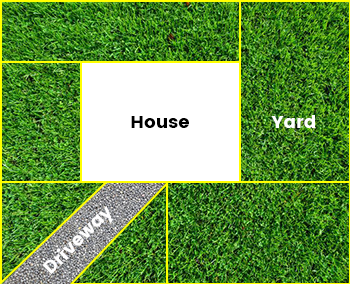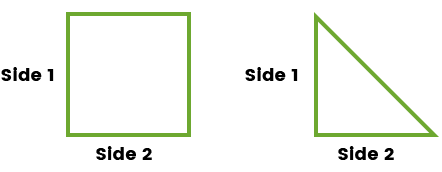#### Measuring

Brookmeade will guide you through the measuring process, call in with measurements and we will give you all your options. Call 804-883-6338.

The grid below can help you determine the amount of sod you need. Many yards are an odd shaped, do your best to square it off and measure each area separately.

To get an idea of how much sod you will need, think of the area to be sodded as separate shapes like rectangles and triangles. Move the mouse over this diagram for an example:##### Area Estimate Calculator

First measure the sides of the rectangles and triangles that make up the area you want to sod. Then enter the measurements into the calculator below, one area at a time. Click “Add Area” to add each to the sum of areas shown at right. Your estimated total area will be shown in the box

Remember, this is an estimate only.

To do a “Running Yard Measurement” Divide length needed by 6.75 to get number of yards required.

If you wanted to know how much sod is needed for 1 row of 200 feet long, divide 200 by 6.75 = 29.62 or 30 yards.